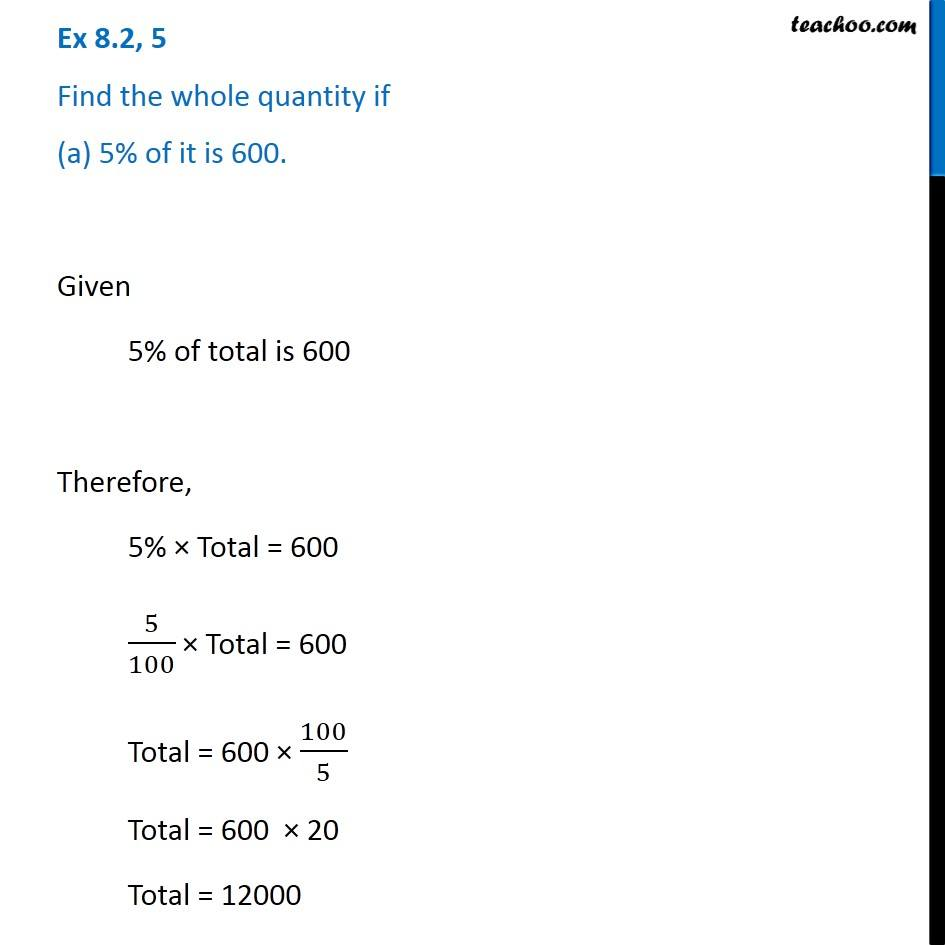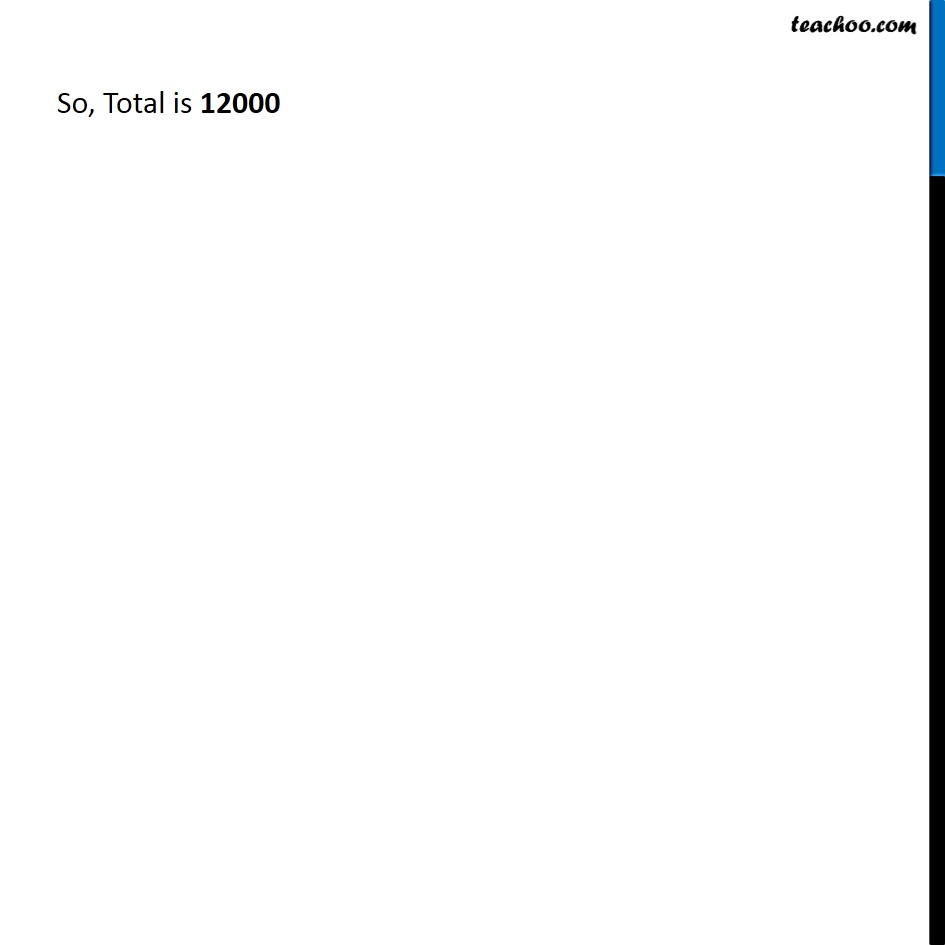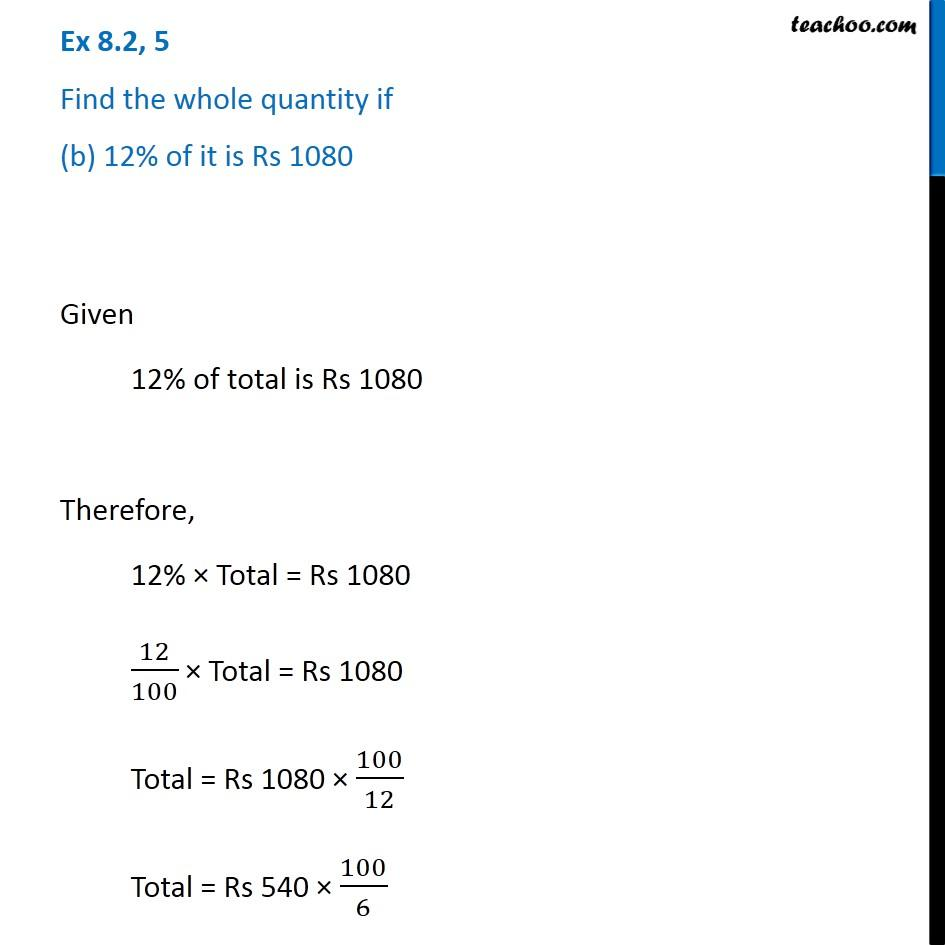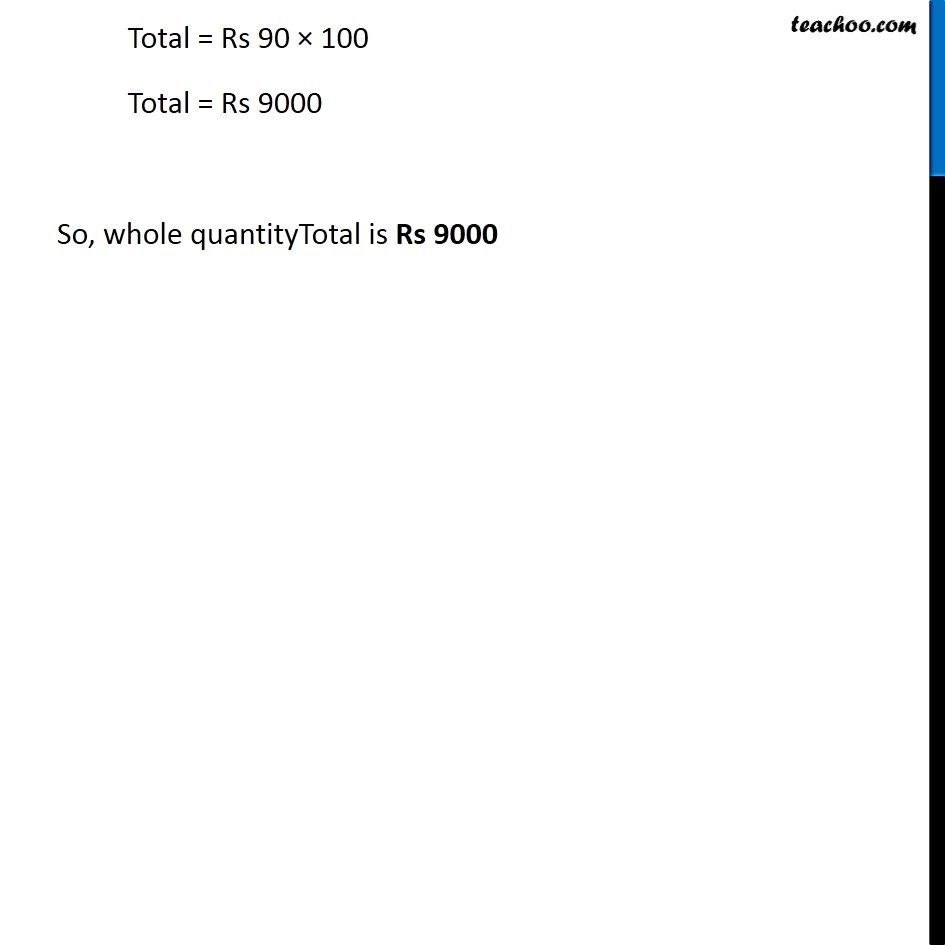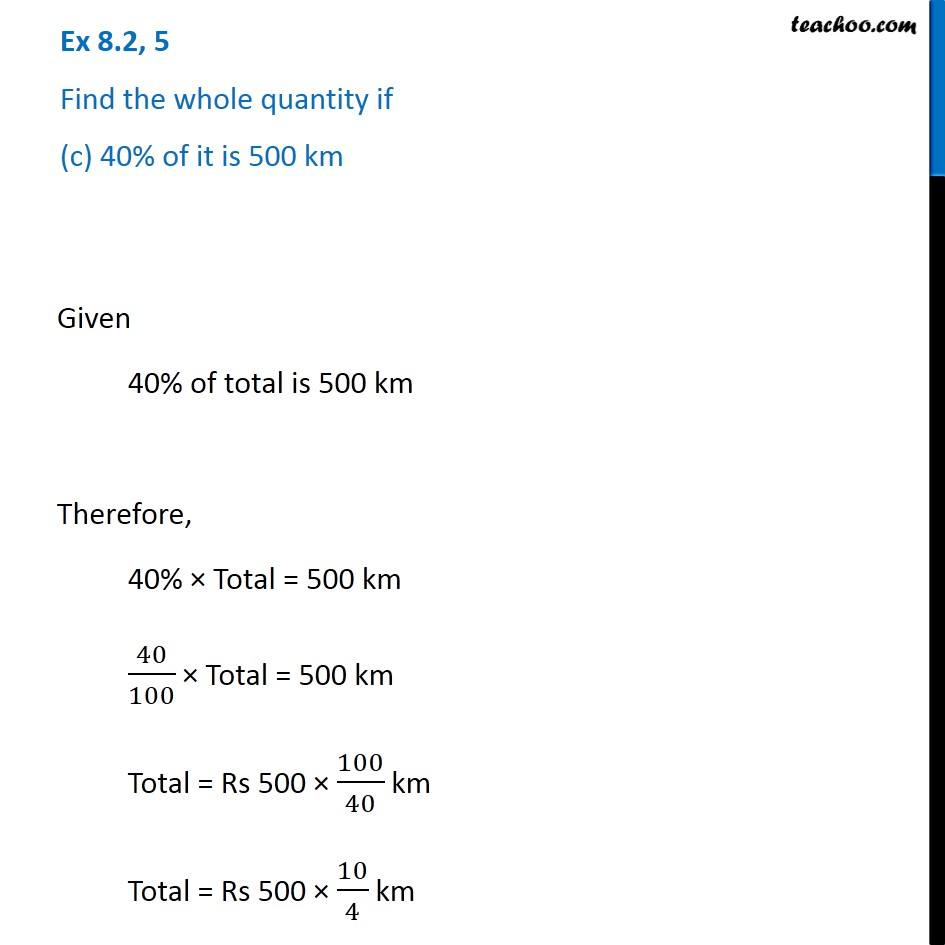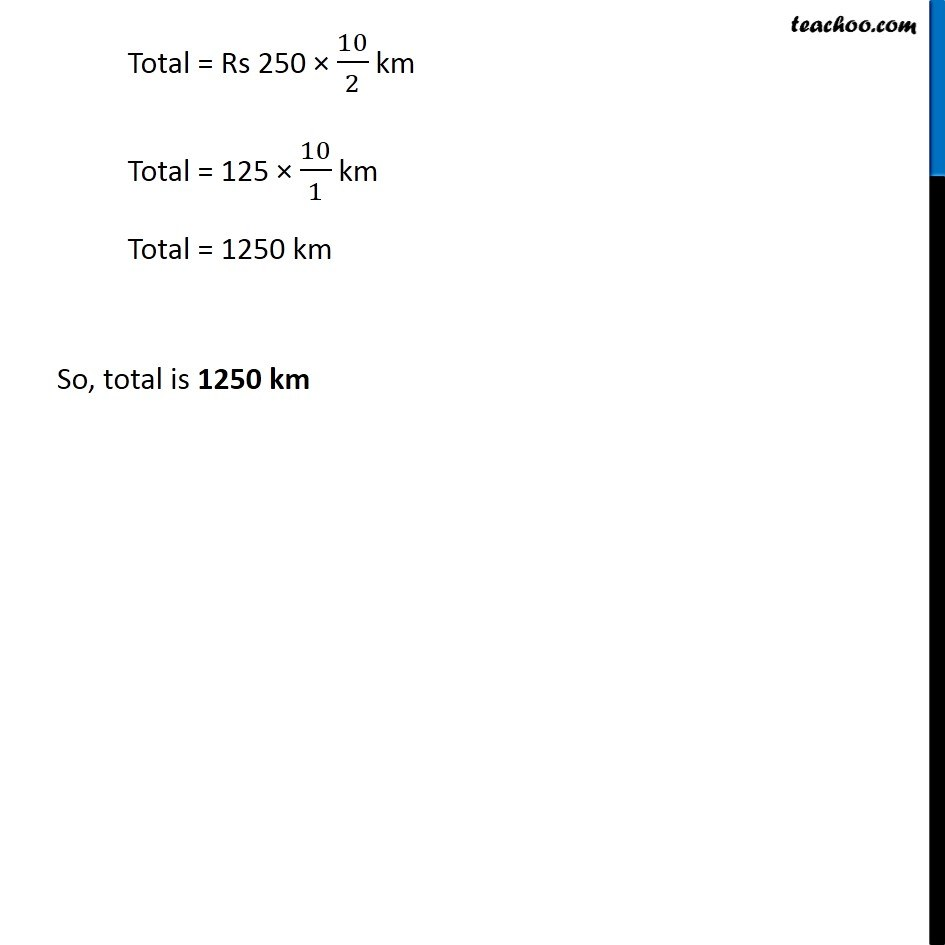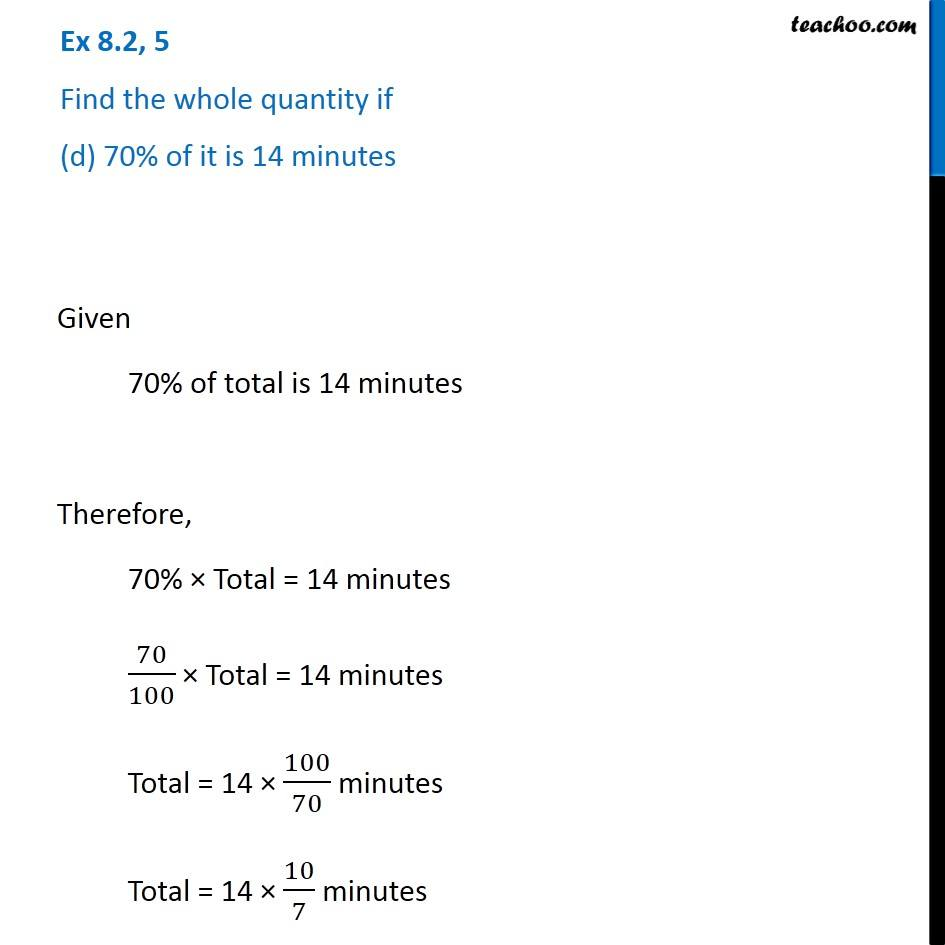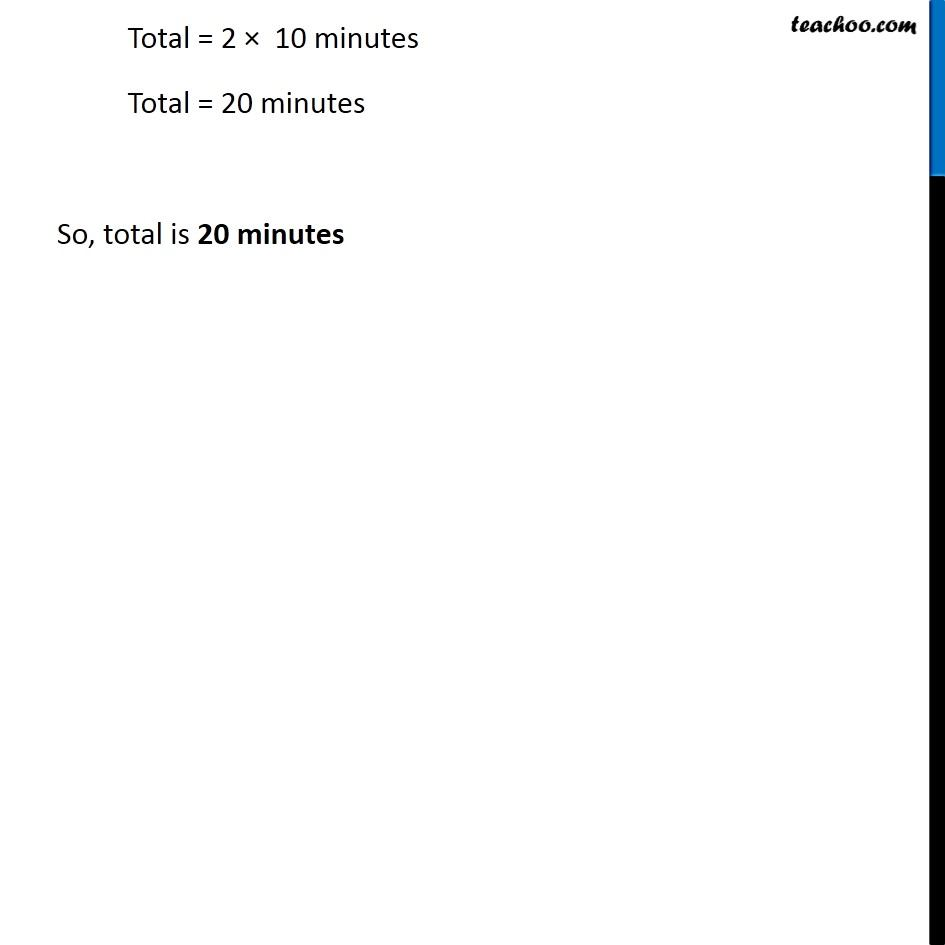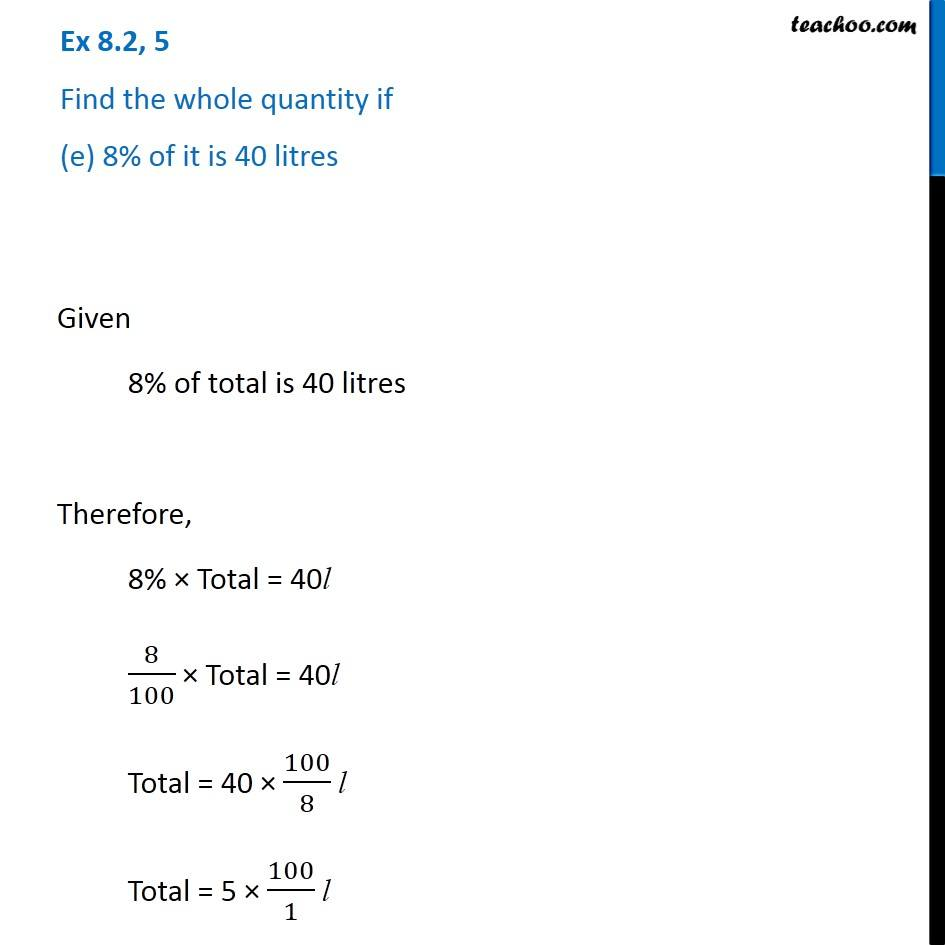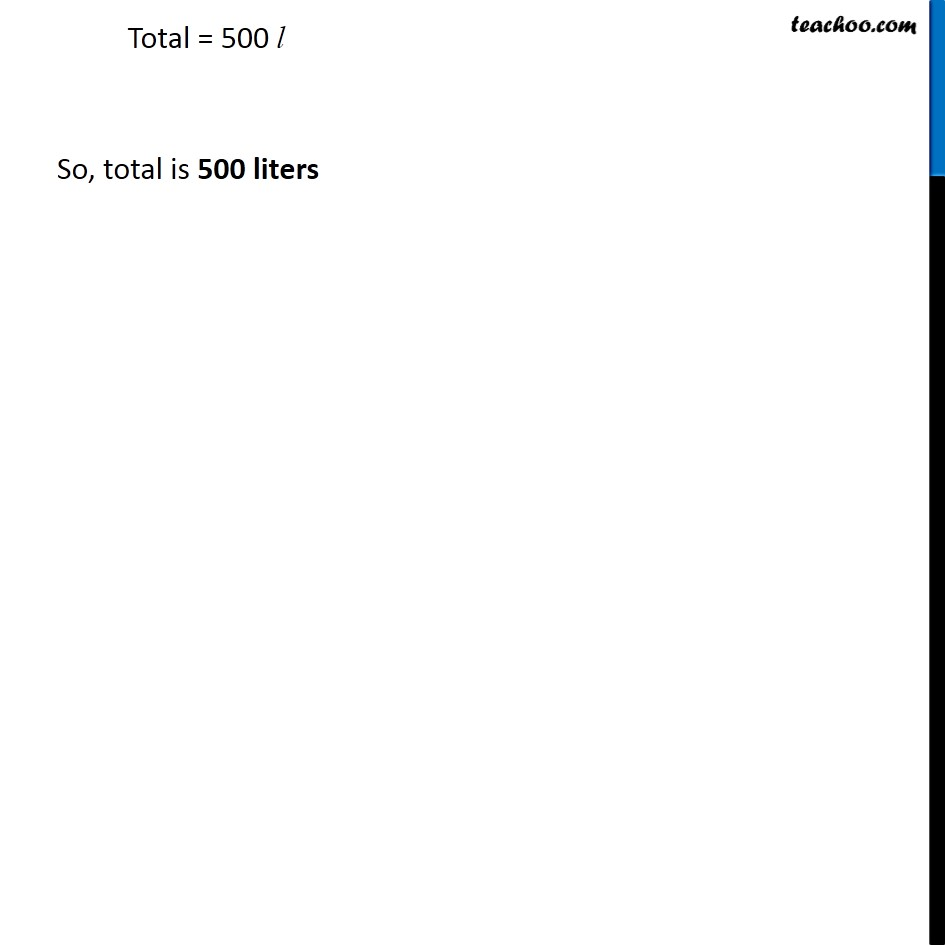Subscribe to our Youtube Channel - https://you.tube/teachoo

1. Chapter 8 Class 7 Comparing Quantities
2. Serial order wise
3. Ex 8.2

Transcript

Ex 8.2, 5 Find the whole quantity if (a) 5% of it is 600. Given 5% of total is 600 Therefore, 5% × Total = 600 5/100 × Total = 600 Total = 600 × 100/5 Total = 600 × 20 Total = 12000 So, Total is 12000 Ex 8.2, 5 Find the whole quantity if (b) 12% of it is Rs 1080 Given 12% of total is Rs 1080 Therefore, 12% × Total = Rs 1080 12/100 × Total = Rs 1080 Total = Rs 1080 × 100/12 Total = Rs 540 × 100/6 Total = Rs 90 × 100 Total = Rs 9000 So, whole quantityTotal is Rs 9000 Ex 8.2, 5 Find the whole quantity if (c) 40% of it is 500 km Given 40% of total is 500 km Therefore, 40% × Total = 500 km 40/100 × Total = 500 km Total = Rs 500 × 100/40 km Total = Rs 500 × 10/4 km Total = Rs 250 × 10/2 km Total = 125 × 10/1 km Total = 1250 km So, total is 1250 km Ex 8.2, 5 Find the whole quantity if (d) 70% of it is 14 minutes Given 70% of total is 14 minutes Therefore, 70% × Total = 14 minutes 70/100 × Total = 14 minutes Total = 14 × 100/70 minutes Total = 14 × 10/7 minutes Total = 2 × 10 minutes Total = 20 minutes So, total is 20 minutes Ex 8.2, 5 Find the whole quantity if (e) 8% of it is 40 litres Given 8% of total is 40 litres Therefore, 8% × Total = 40l 8/100 × Total = 40l Total = 40 × 100/8 l Total = 5 × 100/1 l Total = 500 l So, total is 500 liters

Ex 8.2